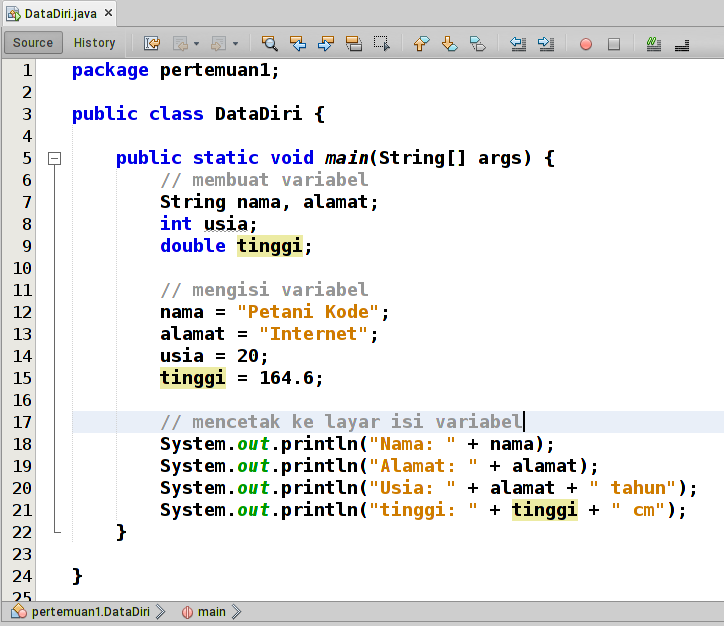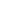| lesson material | material summary | questions and answers | definitions | types and examples | other information | materi pelajaran | ringkasan materi | pertanyaan dan jawaban | definisi | jenis-jenis dan contoh-contoh | informasi lainnya |

# Learning Java Programming: Variables and Data TypesA variable is a place to store temporary values.
That is all.
Serious?
Yeah seriously, if you want a longer one ...
... let's see the meaning from Wikipedia:
Variable: (Lat) 1. changeable, not fixed; 2. declaration of something that has a variety of values ​​3. different in programming languages ​​also called symbols that represent certain values, variables known in sub-programs are called local variables. while what is generally known in one program is called a global variable.
Well, do you understand?
If not, understand enough: Variables as a place to store temporary values.
Then, what is the data type?
Data type is a type of data stored in a variable.

## Types of Data Types

The following are various types of data in Java:
• `char`: Character data type, example `Z`
• `int`: numbers or integers, for example `29`
• `float`: decimal number, for example `2.1`
• `double`: decimal number too, but more capacity, for example `2.1`
• `String`: a collection of characters that make up text, for example `Hello World!`
• `boolean`: data types that are only valuable `true`and`false`

## Making Variables

The thing you need to know in making variables in java is how to write them.
Format it like this:
``````<tipe data> namaVariabel;
``````
Example :
Create an empty variable of type integer:
``````int namaVariabel;
``````
Creates an integer type variable and is immediately filled with a value:
``````int namaVariabel = 19;
``````
Create a set of variables whose data types are the same:
``````int a, b, c;
``````
Then, where was it written?
1. Inside functions `main()`: variables written in other functions `main()`and functions are called local variables.
2. In the class: This variable is called a class or global variable.
Confused…?

## Let's Try Exercises by Creating a DataDiri Program

Create a new program called DataDiri. Please add a class (java class) just package (package) `pertemuan1` .In the window that appears, fill in the name of the class with DataDiri . After that, please follow the following code.After that, try running by pressing the [Shift]button [F6]Analyze and understand the intent of the codes above.The output seems wrong.
How old is the address, why is that?
That is because we call the variable `alamat`at the age line .
So what will appear is the contents of the variable `alamat`, not the contents of the variable `usia`.
Repair the code to be like this:And, ... please try running the program again.Yep! the result is correct.

## Variable Writing Rules

Apparently not allowed to create variables.
There are rules that must be followed, including:
• The variable name may not use the keywords of the Java (reserved word) such as `if``for``switch`, etc.
• Variable names may use letters, numbers (0-9), underscore , and dollar symbols (\$), but the use of underscores and symbols is better avoided.
• The variable name must start with a lowercase letter, because Java uses the CamelCase style .
• If the variable name is more than 1 syllable, then the second word is written by starting with a capital letter and so on, for example `namaVariabel`.

## 2nd Exercise: Broad Circle Program

Let us establish understanding by making a Broad Circularprogram .
This program functions to calculate the area of ​​a circle.
We can calculate the area of ​​a circle using the formula .`PI x r2`
Before starting to program, we should first understand the algorithm and flowchart:

### Algorithm

``````Deklarasi:
Double luas, PI
int r
Deskripsi:
- Input
PI = 3.14
r = 18
- Proses
luas = PI * r * r
- Output
cetak luas
``````

### Flow chart:Well, it's clear right?
Now we are just coding .Please execute and refine the results.

## Data Type Conversion

Conversion means changing to another type.
Why do we need to convert data types?
To answer this, I want to show the following illustration:

Water that is in liquid form cannot be stored in cardboard. Therefore, water must be converted into a solid form (ice) so that it can be stored in cardboard.
Likewise with variables.
Data string types can not be stored in variables with integertypes .

### How to Convert Data Types

Example: Convert to integer type
Method 1:
``````Integer.perseInt(variabel);
Integer.perseInt(1.2);
``````
Method 2:
``````Integer.valueOf(2.1);
``````
Method 3:
``````objek.toInt();
``````
Method 4:
``````(int) variabel;
(int) 2.1;
``````
Apparently, there are many ways.

### Let's Try in the Program ...

Make a new class (java class) in the meeting package2 .
Give a name: Extensive Triangle .
Then follow the following program code:
``````package pertemuan2;

import java.util.Scanner;

public class LuasSegitia {

public static void main(String[] args) {
// deklarasi
Double luas;
int alas, tinggi;

// mebuat scanner baru
Scanner baca = new Scanner(System.in);

// Input
System.out.println("== Program hitung luas Segitiga ==");
System.out.print("Input alas: ");
alas = baca.nextInt();
System.out.print("Input tinggi: ");
tinggi = baca.nextInt();

// proses
luas = Double.valueOf((alas * tinggi) / 2);

// output
System.out.println("Luas = " + luas);
}

}
``````
Variables of `luas`type Double data , meaning the value that can be stored is Double .
While the variables `alas`and `tinggi`types are Integer .
In order for the results of integer data operations to be stored in a variable of type double, it needs to be converted.
If executed, it will produce:##### 0 Komentar untuk "Learning Java Programming: Variables and Data Types"

Silahkan berkomentar sesuai artikel

Template By Kunci Dunia